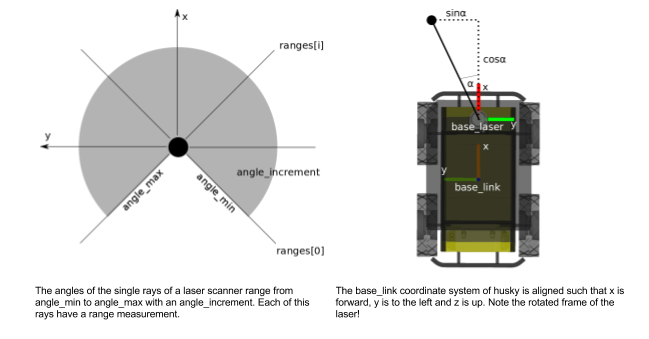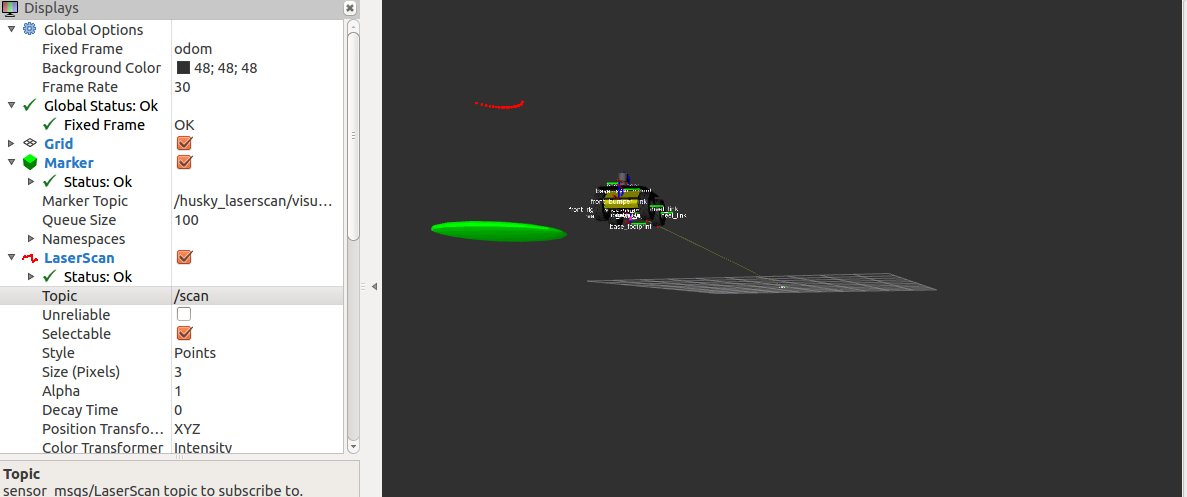A compilation of concepts I want to remember...

Navigation
» Home
» About Me
» Github

# ROS+RViz: Husky p-controller #1

14 Mar 2017 » ros

A simple proportional controller implemented in C++ using ROS and simulated in Gazebo with visualization done through Rviz…package available here.

This is a result of working through exercise 3, provided by the introductory course on ROS at ETZH. My personal opinion is that this is one of the most clear and concise introductions that I have come across, and also provides a guide post on best practices, which I surely will look to implement in my own work.

The p-controller, is a control that simply adjusts velocity in proportion to the distance to the target, in this case the pillar. Despite the controller working, I had to make some on-the-fly hacks.The main factors that need to be resolved:

1. Distance to Pillar. Since the pillar is the only object in the world, problem clearly simplified for us (thanks!), the distance is simply the minimum range that the laser scan returns and is found in ranges[].

2. Orientation of Pillar from the perspective of the base_laser (see above figure) is alpha, and can be determined quickly, as in the discovery of the minimum distance, we also get the index, or number of angle_increments for free, and can use this in calculating the orientation.

3. The linear velocity in x and y direction, was set as to be the distance to the pillar, thus the velocity would decrease as the proximity to the Pillar increased. Further, to avoid a collision, the velocity was set to 0, if Husky was within 0.5m of the pillar. The linear velocity and angular velocity (which is was simply set to alpha), is pumped into a geometry_msgs::Twist message and published for listening by gazebo and Rviz.

4. In order to place the marker in the correct position (here is a tutorial on markers btw), we need the cartesian coordinates, which again can be quickly determined with some trivial geometry. The x, y coordinates are then set in visualization_msgs::Marker message and subsequently published using the appropriate publisher.

Thats really it…and you get a pretty cool looking simulation running. This is a snapshot of Husky running in RViz, with laser scan, marker, robot model, and tf all set accordingly.